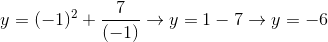# High School Math : Understanding Domain and Range

## Example Questions

### Example Question #1 : Understanding Domain And Range

What is the domain of the function?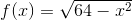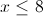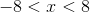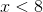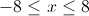Explanation:

The domain of a function is the set of possible values for the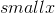variable. The range would be the possible values for the solution,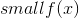.

The value inside of a square root must be greater than or equal to zero in order to have a real solution.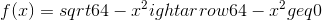Now we can solve forin the inequality.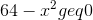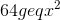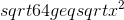Square roots have positive and negative roots, so we need to set up two results. Remember to switch the direction fo the inequality for the negative solution.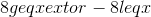These solutions can be combined to give our final answer.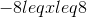Any values ofthat are not included will result in an imaginary (impossible) answer.

### Example Question #2 : Understanding Domain And Range

Which of the following does NOT belong to the domain of the function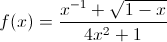?

1/2

-1

-1/2

0

1

0

Explanation:

The domain of a function includes all of the values of x for which f(x) is real and defined. In other words, if we put a value of x into the function, and we get a result that isn't real or is undefined, then that value won't be in the domain.

If we let x = 0, then we will be forced to evaluate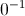, which is equal to 1/0. The value of 1/0 is not defined, because we can never have zero in a denominator. Thus , because f(0) isn't defined, 0 cannot be in the domain of f(x).

### Example Question #3 : Understanding Domain And Range

What is the domain of the function: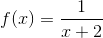All integers.

All positive numbers.

All real numbers except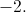All real numbers except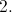All real numbers.

All real numbers exceptExplanation:

The domain of a function consists of all of the possible values for x. In this case, we want to make sure that we are not dividing by(in the denominator), since that would make our function undefined. Having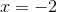would make the denominator. Thus, that is not in our domain. There is nothing else that would make this function undefined, and thus the domain is all real numbers except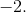### Example Question #4 : Understanding Domain And Range

What is the range of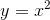?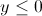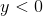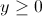All real numbers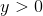Explanation:

The range of a function is all of the possiblevalues that the equation can take. For this equation ourvalue cannot be negative, as a negative number squared still gives us a positive value.

Since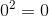, we know that the lowest possible value thatcan reach is. Therefore the range is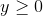.

### Example Question #5 : Understanding Domain And Range

Find the domain of the following function.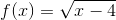All real numbers exceptAll real numbers such that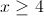.

All real numbers except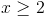All real numbers such that.

Explanation:

Domain represents all possible values for. In this situation, we want to make sure that the numbers under the square root are greater than or equal to 0. This will be the case when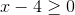. Thus, the domain includes all real numbers such that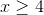### Example Question #6 : Understanding Domain And Range

What is the range of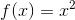?

All real numbers

All rational numbers

All real numbers greater than or equal to 0

All real numbers greater than 0

All real numbers except -1

All real numbers greater than or equal to 0

Explanation:

The range of a function is defined as the possible values for, or the possible outcomes. In this function, it is not possible to get any sort of negative number as an outcome. It is possible to get zero when. Thus, the range is all real numbers greater than or equal to 0.

### Example Question #1 : Functions And Graphsis a sine curve. What are the domain and range of this function?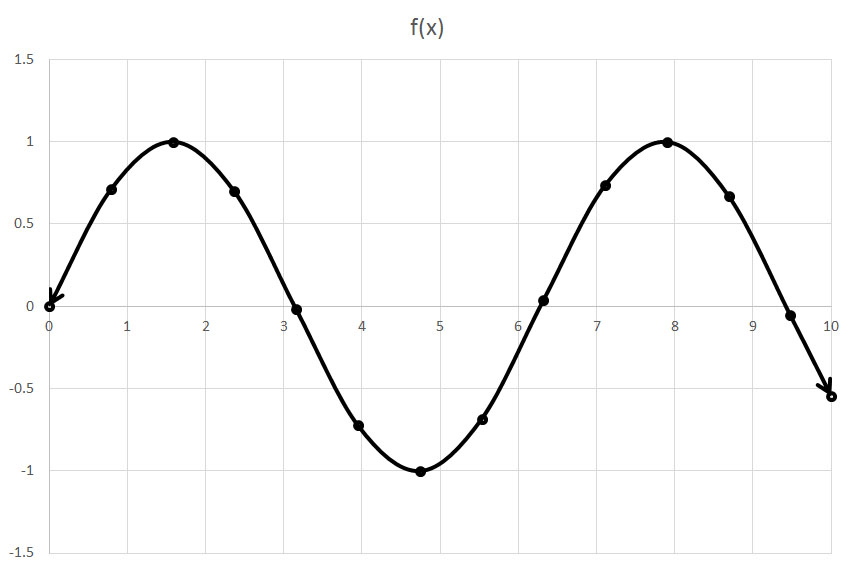Domain: All real numbers

Range: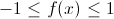Domain: All real numbers

Range: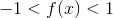Domain: All real numbers

Range:Explanation:

The domain includes the values that go into a function (the x-values) and the range are the values that come out (theor y-values). A sine curve represent a wave the repeats at a regular frequency. Based upon this graph, the maximumis equal to 1, while the minimum is equal to –1. The x-values span all real numbers, as there is no limit to the input fo a sine function. The domain of the function is all real numbers and the range is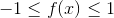.

### Example Question #6 : Domain And Range

If, which of these values ofis NOT in the domain of this equation?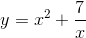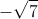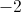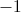Usingas the input () value for this equation generates an output () value that contradicts the stated condition of.
Thereforeis not a valid value forand not in the equation's domain: